# Math in Focus Grade 1 Chapter 17 Practice 4 Answer Key Subtraction with Regrouping

Go through the Math in Focus Grade 1 Workbook Answer Key Chapter 17 Practice 4 Subtraction with Regrouping to finish your assignments.

## Math in Focus Grade 1 Chapter 17 Practice 4 Answer Key Subtraction with Regrouping

Subtract.

Question 1.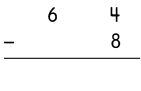64 – 8 = 56.

Explanation:
Here, we will perform regrouping as the number 4 is less than 8. Regrouping is defined as the process of making groups of tens when carrying out operations like addition and subtraction with two-digit numbers or larger numbers. So the subtraction of 64-8 is 56.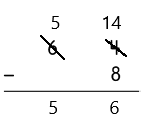Question 2.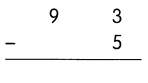93-5 = 88.

Explanation:
Here, we will perform regrouping as the number 3 is less than 5. Regrouping is defined as the process of making groups of tens when carrying out operations like addition and subtraction with two-digit numbers or larger numbers. So the subtraction of 93-5 is 88.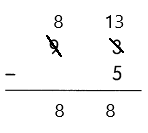Question 3.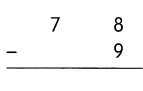78-9 = 69.

Explanation:
Here, we will perform regrouping as the number 8 is less than 9. Regrouping is defined as the process of making groups of tens when carrying out operations like addition and subtraction with two-digit numbers or larger numbers. So the subtraction of 78-9 is 69.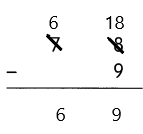Question 4.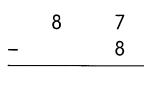87-8 = 79.

Explanation:
Here, we will perform regrouping as the number 7 is less than 8. Regrouping is defined as the process of making groups of tens when carrying out operations like addition and subtraction with two-digit numbers or larger numbers. So the subtraction of 87-8 is 79.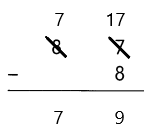Question 5.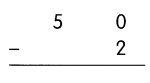50-2 = 48.

Explanation:
Here, we will perform regrouping as the number 0 is less than 2. Regrouping is defined as the process of making groups of tens when carrying out operations like addition and subtraction with two-digit numbers or larger numbers. So the subtraction of 50-2 = 48.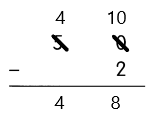Question 6.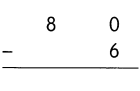80-6 = 74.

Explanation:
Here, we will perform regrouping as the number 0 is less than 6. Regrouping is defined as the process of making groups of tens when carrying out operations like addition and subtraction with two-digit numbers or larger numbers. So the subtraction of 80-6 is 74.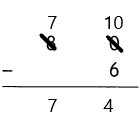Fill in the missing numbers.

Question 7.
72 – 9 = _________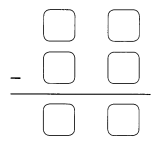72-9 = 63.

Explanation:
Here, we will perform regrouping as the number 2 is less than 9. Regrouping is defined as the process of making groups of tens when carrying out operations like addition and subtraction with two-digit numbers or larger numbers. So the subtraction of 72-9 is 63.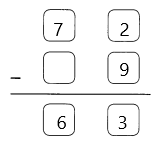Question 8.
91 – 4 = ___________91-4 = 87.

Explanation:
Here, we will perform regrouping as the number 1 is less than 4. Regrouping is defined as the process of making groups of tens when carrying out operations like addition and subtraction with two-digit numbers or larger numbers. So the subtraction of 91-4 is 87.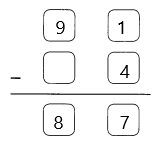Question 9.
Kayla drops a ball into a number machine. Which ball is it? Write the number in the ○.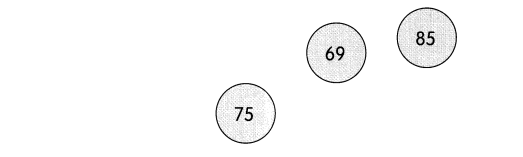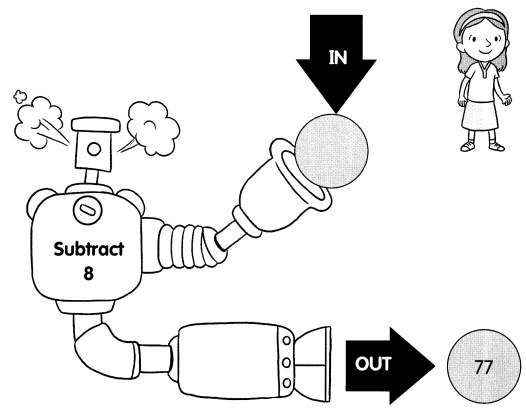The ball is 85.

Explanation:
Given that Kayla drops a ball into a number machine, it subtracts 8 and the output is 77. So to find the ball we will add 77+8 which is 85. So the ball is 85.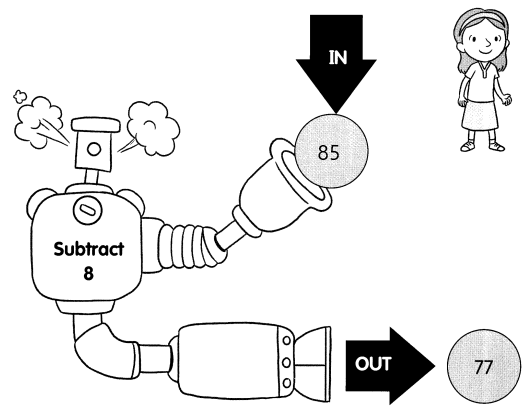Subtract.

Question 10.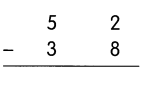52-38 = 14.

Explanation:
Here, we will perform regrouping as the number 2 is less than 8. Regrouping is defined as the process of making groups of tens when carrying out operations like addition and subtraction with two-digit numbers or larger numbers. So the subtraction of 52-38 is 14.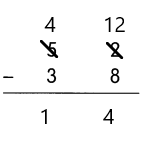Question 11.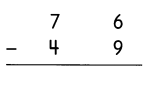76-49 = 27.

Explanation:
Here, we will perform regrouping as the number 6 is less than 9. Regrouping is defined as the process of making groups of tens when carrying out operations like addition and subtraction with two-digit numbers or larger numbers. So the subtraction of 76-49 is 27.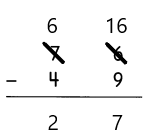Question 12.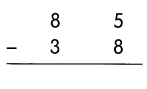85-38 = 47.

Explanation:
Here, we will perform regrouping as the number 5 is less than 8. Regrouping is defined as the process of making groups of tens when carrying out operations like addition and subtraction with two-digit numbers or larger numbers. So the subtraction of 85-38 is 47.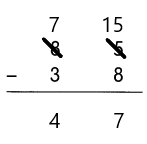Question 13.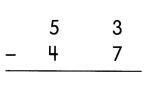53-47 = 6.

Explanation:
Here, we will perform regrouping as the number 3 is less than 7. Regrouping is defined as the process of making groups of tens when carrying out operations like addition and subtraction with two-digit numbers or larger numbers. So the subtraction of 53-47 is 6.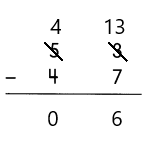Question 14.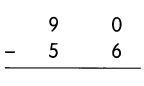90-56 = 34.

Explanation:
Here, we will perform regrouping as the number 0 is less than 6. Regrouping is defined as the process of making groups of tens when carrying out operations like addition and subtraction with two-digit numbers or larger numbers. So the subtraction of 90-56 is 34.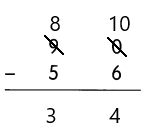Question 15.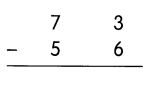73-56 = 17.

Explanation:
Here, we will perform regrouping as the number 3 is less than 6. Regrouping is defined as the process of making groups of tens when carrying out operations like addition and subtraction with two-digit numbers or larger numbers. So the subtraction of 73-56 is 17.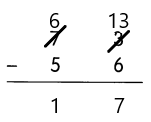Fill in the missing numbers.

Question 16.
83 – 26 = __________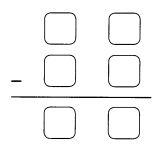83-26 = 57.

Explanation:
Here, we will perform regrouping. Regrouping is defined as the process of making groups of tens when carrying out operations like addition and subtraction with two-digit numbers or larger numbers. So the subtraction of 83-26 is 57.

Question 17.
95 – 38 = ____________95-38 = 57.

Explanation:
Here, we will perform regrouping. Regrouping is defined as the process of making groups of tens when carrying out operations like addition and subtraction with two-digit numbers or larger numbers. So the subtraction of 95-38 is 57

Question 18.
Ken drops a ball into a number machine. Which ball is it? Write the number in the ○.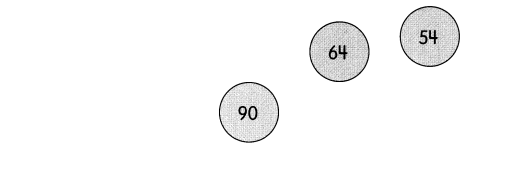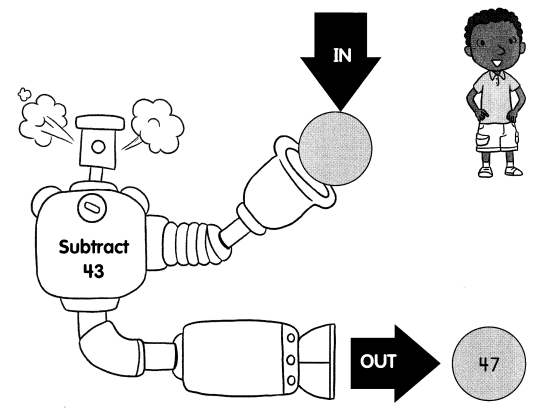The in ball is 90.

Explanation:
Gien that Ken drops a ball and it subtracts 43. The out ball is 47, so the in ball is 47+43 which is 90.

Question 19.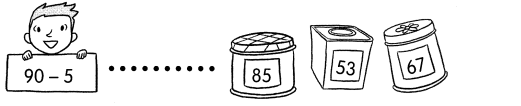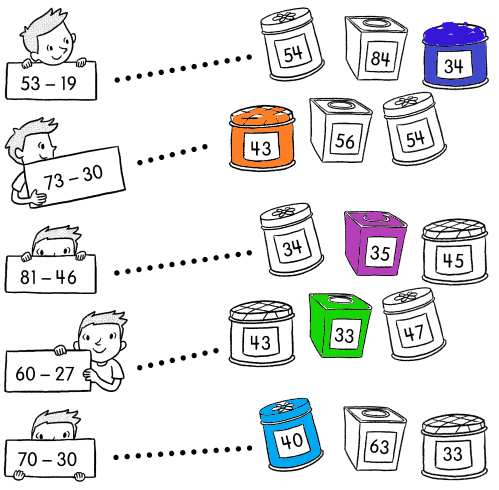Subtract.

Question 20.
56 – 8 = __________
56-8 = 48.

Explanation:
Here, we will perform regrouping. Regrouping is defined as the process of making groups of tens when carrying out operations like addition and subtraction with two-digit numbers or larger numbers. So the subtraction of 56-8 is 48.

Question 21.
73 – 4 = __________
73-4 = 69.

Explanation:
Here, we will perform regrouping. Regrouping is defined as the process of making groups of tens when carrying out operations like addition and subtraction with two-digit numbers or larger numbers. So the subtraction of 73-4 is 69.

Question 22.
67 – 8 = ___________
67-8 = 59.

Explanation:
Here, we will perform regrouping. Regrouping is defined as the process of making groups of tens when carrying out operations like addition and subtraction with two-digit numbers or larger numbers. So the subtraction of 67-8 is 59.

Question 23.
75 – 9 = ____________
75-9 = 66.

Explanation:
Here, we will perform regrouping. Regrouping is defined as the process of making groups of tens when carrying out operations like addition and subtraction with two-digit numbers or larger numbers. So the subtraction of 75-9 is 66.

Question 24.
50 – 40 = __________

Explanation:
Here, we will perform regrouping. Regrouping is defined as the process of making groups of tens when carrying out operations like addition and subtraction with two-digit numbers or larger numbers. So the subtraction of

Question 25.
87 – 11 = ___________
87-11 = 76.

Explanation:
The subtraction of 87-11 is 76.

Question 26.
90 – 50 = ___________
90-50 = 40.

Explanation:
The subtraction of 90-50 is 40.

Question 27.
93 – 20 = __________
93-20 = 73.

Explanation:
The subtraction of 93-20 is 73.

Question 28.
58 – 18 = __________
58-18 = 40.

Explanation:
The subtraction of 58-18 is 40.

Question 29.
61 – 14 = __________
61-14 = 47.

Explanation:
Here, we will perform regrouping. Regrouping is defined as the process of making groups of tens when carrying out operations like addition and subtraction with two-digit numbers or larger numbers. So the subtraction of 61-14 is 47.

Question 30.
47 – 39 = __________
47-39 = 8.

Explanation:
Here, we will perform regrouping. Regrouping is defined as the process of making groups of tens when carrying out operations like addition and subtraction with two-digit numbers or larger numbers. So the subtraction of 47-39 is 8.

Question 31.
53 – 27 = __________
53-27 = 26.

Explanation:
Here, we will perform regrouping. Regrouping is defined as the process of making groups of tens when carrying out operations like addition and subtraction with two-digit numbers or larger numbers. So the subtraction of 53-27 is 26.

Question 32.
30 – 28 = __________